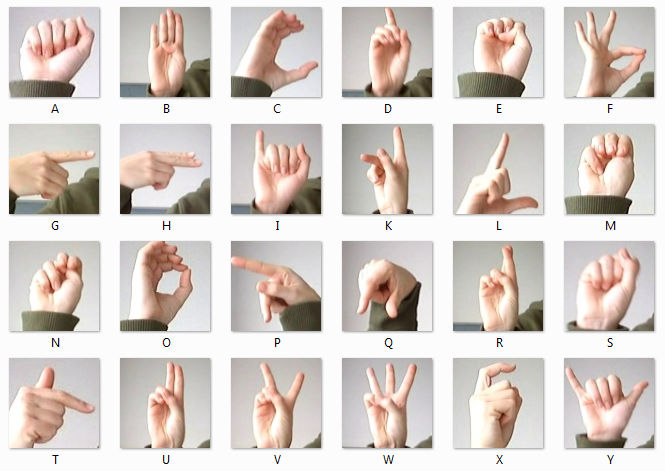# Sign Language Classifier using CNN

Source: Deep Learning on Medium# Sign Language Classifier using CNN

Implementation of a Convolutional Neural Network on the MNIST sign language dataset.

# 1. The dataset

The MNIST sign language dataset is avalaible on Kaggle ( https://www.kaggle.com/datamunge/sign-language-mnist )

It is composed of 27 500 training instances and 7000 testing instances.

Each instance is a 24×24 pixel image, converted into an array of 255 values so that each image can be read as a 1-D array. Here is a picture of all the signs with it’s corresponding letters :

# 2. Frame the problem

The objective of this project is to label an image of a sign to it’s corresponding letter.

This type of problem is a supervised problem because every feature has it’s associated label. Also, it is a classification task because all the labels values are discrete (from A to Z, excluding J and Z because it requires motion).

This dataset was created so it can be hard to solve using standart machine learning techniques. This is due to the fact that the images has complex shapes and contours.

We will be using a Convolutional Neural Network in order to solve this problem because it perform well on finding common pattern in complex images.

# 3. Data exploration

In order to load the data we will be using Pandas.

We can use built in Seaborn function countplot() to show the number of observations in each category.

The bar plot shows us that the dataset is equally distributed, so we do not have to resample the dataset.

# 4. Prepare the data

4.1 Converting DataFrame into Numpy arrays

We will be converting our DataFrame records into Numpy arrays. That way we will have one 1D array per image instead of a dataframe record with 255 columns.

4.2 Creating a validation dataset

We will split the training dataset into 2 datasets, one for training and the other for validation. We will use 80% of the dataset for training, and 20% for testing. Scikit Learn built in function train_test_split does that for us :

4.3 Normalize the data

In any Machine Learning or Deep Learning project, it is always indispensable to normalize the data. The reason behind is that it will help the model compute the weights. In order to do that we just have to divide each values by 255, since the value range is between 0 and 255.

4.4 Reshape the labels

Since we are dealing with categorical data, it is important to reshape the labels using One-Hot Encoding. The reason behind is that the model will assume that there is a natural ordering between categories and it will result in poor performances and unexpected results. One-Hot encode will replace each label with a binary array. That array will be the size of all possible label values (24). All values will be assign to 0 except the one that corresponds to the instance. We can use Scikit-Learn built-in function LabelBinarizer in order to do that